## RD Sharma Class 10 Solutions Chapter 4 Quadratic Equations Ex 4.4

These Solutions are part of RD Sharma Class 10 Solutions. Here we have given RD Sharma Class 10 Solutions Chapter 4 Quadratic Equations Ex 4.4

Other Exercises

Find the roots of the following quadratic equations (if they exist) by the method of completing the square.
Question 1.
x² – 4 √2x + 6 = 0
Solution: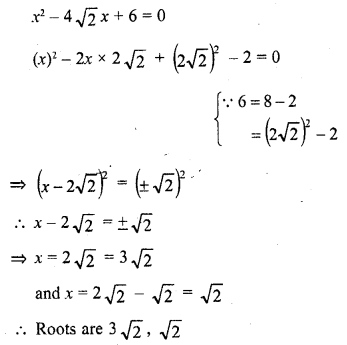Question 2.
2x² – 7x + 3 = 0
Solution: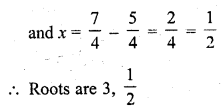Question 3.
3x² + 11x + 10 = 0
Solution: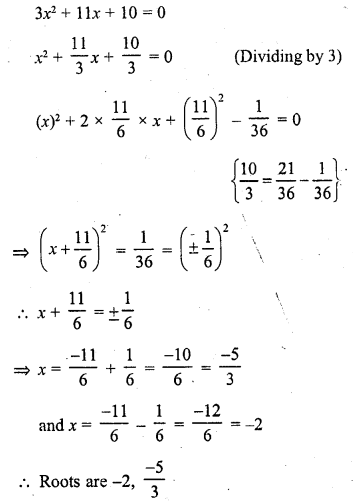Question 4.
2x² + x – 4 = 0
Solution: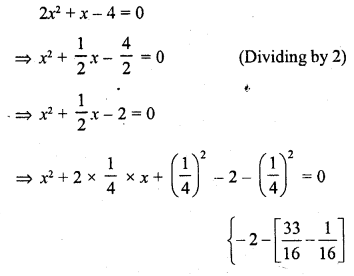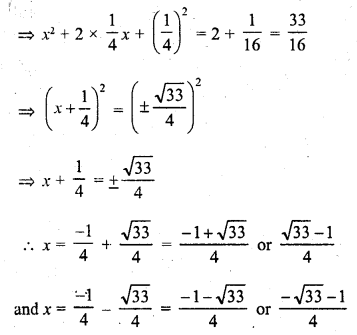Question 5.
2x² + x + 4 = 0
Solution: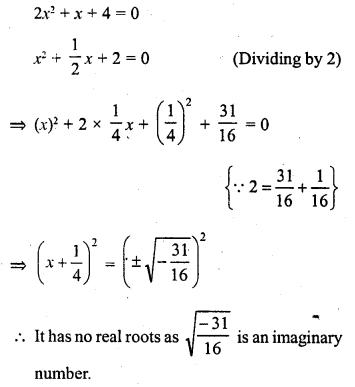Question 6.
4x² + 4√3x + 3 = 0
Solution:Question 7.
√2 x² – 3x – 2√2 = 0
Solution: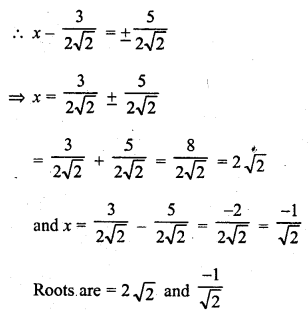Question 8.
√3 x² + 10x + 7√3 = 0
Solution:Question 9.
x² – (√2 + 1)x + √2 = 0
Solution: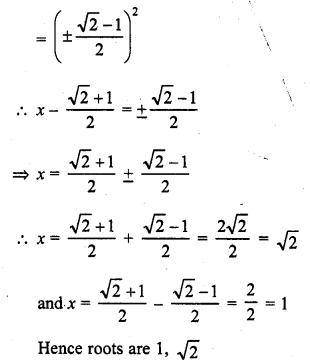Question 10.
x² – 4ax + 4a² – b² = 0
Solution: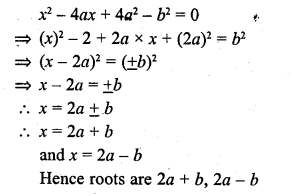Hope given RD Sharma Class 10 Solutions Chapter 4 Quadratic Equations Ex 4.4 are helpful to complete your math homework.

If you have any doubts, please comment below. Learn Insta try to provide online math tutoring for you.